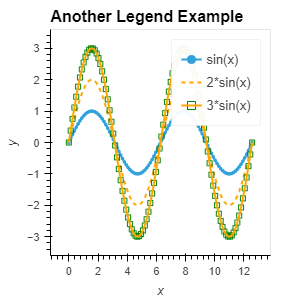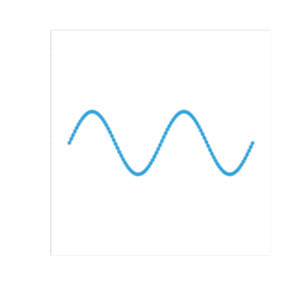# Strange behavior in legend when curve line_dash not 'solid'

I have noticed strange behavior in a legend if it contains a curve with a line_dash set to anything other than ‘solid’. In the below code, from the docs https://holoviews.org/gallery/demos/bokeh/legend_example.html when you click on the legend for 2*sin(x) it will disappear! If you set line_dash=‘solid’ it will only become muted, as is the behavior for the other curves.

Is this the desired behavior or is it a bug?

Original appearance:Becomes after clicking on 2*sin(x) in legend:import numpy as np
import holoviews as hv
hv.extension(‘bokeh’)

x = np.linspace(0, 4*np.pi, 100)
y = np.sin(x)

scatter1 = hv.Scatter((x, y), label=‘sin(x)’)
scatter2 = hv.Scatter((x, y2), label='2sin(x)‘).opts(color=‘orange’)
scatter3 = hv.Scatter((x, y3), label='3sin(x)’).opts(color=‘green’)
scatter4 = hv.Scatter(scatter3).opts(line_color=‘green’, marker=‘square’, fill_alpha=0, size=5)

curve1 = hv.Curve(scatter1)
curve2 = hv.Curve(scatter2).opts(line_dash=(4, 4), color=‘orange’)
curve3 = hv.Curve(scatter3).opts(color=‘orange’)

example2 = scatter1 * curve1 * curve2 * scatter4 * curve3

example2.relabel(“Another Legend Example”)

This is a bug in Bokeh and will be fixed in Bokeh 3.3 ref.

If you need this behavior right now, you can run `hv.renderer("bokeh").webgl = False`.

1 Like

Thank you! It took me hours to identify in one of my plots what triggered the issue.